# Basic functions - math word problems

1. Roses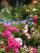On the large rosary was a third white, half red, yellow quarter and six pink. How many roses was in the rosary?
2. Sum and roundingI know two numbers whose sum is 20. When they each round and then added together I get the 30. What are this numbers?
3. Three piles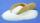100 kg of sugar we divide into three piles. The first pile is small. If we added to second 2 kg of sugar it would have 25% more sugar than the first pile. If we added to third pile 3 kg of sugar it would have 20% more sugar than the 2nd pile. How many ki
4. Is right triangleOne angle of the triangle is 36° and the remaining two are in the ratio 3:5. Determine whether triangle is a rectangular triangle.
5. Drying apples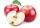By drying, apples lose 85% of their weight. How many kgs of dried apples do we get from 820 kilograms of fresh?
6. Tablecloth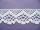The table top is 90 cm long and 50 cm wide. How many cm lace edging is necessary for the tablecloth that hangs on each side by 10 cm?
7. Percentage and rectangleAbout what percentage increases perimeter and area of a rectangle if both the sides 12 cm and 10 cm long we increase by 20%?
8. PercentCalculate how many % is the number 26.25 less than the number 105.
9. Numbers at ratio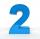The two numbers are in a ratio 3:2. If we each increase by 5 would be at a ratio of 4:3. What is the sum of original numbers?
10. Hexagon = 8 parts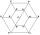Divide the regular hexagon into eight equal parts.
11. AgesJohn, Teresa, Daniel and Paul have summary 56 years. Their ages are in a ratio of 1:2:5:6. Determine how many years have each of them.
12. Moneys in triple ratioMilan, John and Lili have a total 344 euros. Their amounts are in the ratio 1:2:5. Determine how much each of them has?
13. Square - increased perimeterHow many times is increased perimeter of the square, where its sides increases by 150%? If the perimeter of square will increase twice, how much% increases the content area of the square?
14. Volume of three cuboids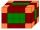Calculate the total volume of all cuboids for which the the size of the edges are in a ratio of 1:2:3, and one of the edges has a size 6 cm.
15. Foot in busIt was 102 people on the bus. 28 girls had two dogs. A 11 girls had one dog. At the next stop seceded 5 dogs (even with their owners). They got two boys together with three dogs. The bus drove one driver. How many foot were in bus?
16. Three drivers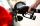Three driversdriving the same direction found that they have same amounth of gasoline. The first is enough to go 6 km, 4 km second and third 3km. Gasoline they divided so all three just drove to the nearest petrol station. How many km away was a petrol st
17. Triangle KLBIt is given equilateral triangle ABC. From point L which is the midpoint of the side BC of the triangle it is drwn perpendicular to the side AB. Intersection of perpendicular and the side AB is point K. How many % of the area of the triangle ABC is area
18. Desribed circle to rectangle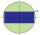Rectangle with sides 6 cm and 4 cm was circumscribed circle. What part of the content of the circle determined by the circumscribed circle occupies a rectangle? Express in perctentages(%).
19. RectangleThe length of the rectangle are in the ratio 5:12 and the circumference is 238 cm. Calculate the length of the diagonal and area of rectangle.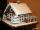Janka and Marienka calculated that there are 210 gingerbreads on the gingerbread house. Janko ate one-seventh of all gingerbreads, and Marienka ate a third less than Janko. How many gingerbreads remained in the gingerbread house?